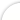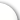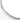Банк рефератов содержит более 364 тысяч рефератов, курсовых и дипломных работ, шпаргалок и докладов по различным дисциплинам: истории, психологии, экономике, менеджменту, философии, праву, экологии. А также изложения, сочинения по литературе, отчеты по практике, топики по английскому.Полнотекстовый поиск.ya-page_js_yes .ya-site-form_inited_no { display: none; }Всего работ: 364139Теги названийРазделыАвиация и космонавтика (304)Административное право (123)Арбитражный процесс (23)Архитектура (113)Астрология (4)Астрономия (4814)Банковское дело (5227)Безопасность жизнедеятельности (2616)Биографии (3423)Биология (4214)Биология и химия (1518)Биржевое дело (68)Ботаника и сельское хоз-во (2836)Бухгалтерский учет и аудит (8269)Валютные отношения (50)Ветеринария (50)Военная кафедра (762)ГДЗ (2)География (5275)Геодезия (30)Геология (1222)Геополитика (43)Государство и право (20403)Гражданское право и процесс (465)Делопроизводство (19)Деньги и кредит (108)ЕГЭ (173)Естествознание (96)Журналистика (899)ЗНО (54)Зоология (34)Издательское дело и полиграфия (476)Инвестиции (106)Иностранный язык (62791)Информатика (3562)Информатика, программирование (6444)Исторические личности (2165)История (21319)История техники (766)Кибернетика (64)Коммуникации и связь (3145)Компьютерные науки (60)Косметология (17)Краеведение и этнография (588)Краткое содержание произведений (1000)Криминалистика (106)Криминология (48)Криптология (3)Кулинария (1167)Культура и искусство (8485)Культурология (537)Литература : зарубежная (2044)Литература и русский язык (11657)Логика (532)Логистика (21)Маркетинг (7985)Математика (3721)Медицина, здоровье (10549)Медицинские науки (88)Международное публичное право (58)Международное частное право (36)Международные отношения (2257)Менеджмент (12491)Металлургия (91)Москвоведение (797)Музыка (1338)Муниципальное право (24)Налоги, налогообложение (214)Наука и техника (1141)Начертательная геометрия (3)Оккультизм и уфология (8)Остальные рефераты (21692)Педагогика (7850)Политология (3801)Право (682)Право, юриспруденция (2881)Предпринимательство (475)Прикладные науки (1)Промышленность, производство (7100)Психология (8692)психология, педагогика (4121)Радиоэлектроника (443)Реклама (952)Религия и мифология (2967)Риторика (23)Сексология (748)Социология (4876)Статистика (95)Страхование (107)Строительные науки (7)Строительство (2004)Схемотехника (15)Таможенная система (663)Теория государства и права (240)Теория организации (39)Теплотехника (25)Технология (624)Товароведение (16)Транспорт (2652)Трудовое право (136)Туризм (90)Уголовное право и процесс (406)Управление (95)Управленческие науки (24)Физика (3462)Физкультура и спорт (4482)Философия (7216)Финансовые науки (4592)Финансы (5386)Фотография (3)Химия (2244)Хозяйственное право (23)Цифровые устройства (29)Экологическое право (35)Экология (4517)Экономика (20644)Экономико-математическое моделирование (666)Экономическая география (119)Экономическая теория (2573)Этика (889)Юриспруденция (288)Языковедение (148)Языкознание, филология (1140)# Реферат: The Photoelectric Effect Essay Research Paper The

 Название: The Photoelectric Effect Essay Research Paper The Раздел: Топики по английскому языку Тип: реферат Добавлен 16:47:30 28 октября 2010 Похожие работы Просмотров: 2 Комментариев: 14 Оценило: 2 человек Средний балл: 5 Оценка: неизвестно     Скачать The Photoelectric Effect Essay, Research Paper The Photoelectric Effect The purpose of this experiment is to determine h/e (Plank’s constant in units of electron volts), the work function F, and the threshold frequency for the photochathode surface provided . Three filters each having a distinct wavelength, were used to carry out this experiment. Utilizing these filters, 435.8 nm, 546.0 nm, and 577.0 nm, data in voltage and current was collected, as potential was selected at random points for each wavelength. This data was then plotted (current vs. potential), and stopping potential was determined. Stopping potential for: 435.8 nm is 1.52 V, 546.0 nm is 1.17 V, and 577.0 nm is 0.8 V. This data was then used to calculate frequency for each wavelength utilizing the equation f = c/l. Frequency data was plotted against stopping potential as mentioned above to determine Plank’s constant, h/e = 3.62 * 10-15 eV*s, also work function, F = 1 V, and threshold frequency, fo = 2.7 * 1014 Hz. Under 12% error is found when compared to the theoretical value (h/e = 4.14 * 10-15 eV*s) for Plank’s constant. This can be a result from the graph, because the best fitting linear line is supposed to be provided in order to calculate Planck’s constant. This has to be very accurate, and the best line was chosen to minimize error. If frequency of light is increased, the maximum KE of the electrons increases linearly, that is KEmax = hf – F. If the frequency, f, is less than the “cutoff” frequency, fo, where hfo = F, no electrons will be emitted. Introduction The photoelectric effect refers to the fact that when light shines in a metal surface, electrons are emitted from the surface . The photoelectric effect occurs in other materials also, but most evident with metals. It is said that electrons should be emitted when light shines on a metal surface is consistent with the electromagnetic wave theory of light, since the electric field of the electromagnetic wave could exert a force on electrons in the metal and thrust some of them out. Einstein pointed out, that the wave theory and the photon theory of light could give very different predictions on the detail of the effect. Examining the wave theory against Einstein’s particle theory. The two important properties of a light wave are its intensity and its frequency (or wavelengths). When these two quantities are varied, the wave theory makes theses following predictions. If light intensity is increased, the number of electrons ejected and their maximum KE should be increased because the higher the intensity means greater electric field amplitude and the greater the electric field should thrust electrons at a higher speed. Also, the frequency of the light should not effect the KE of the electrons. Only the intensity should affect the KE. Do these prediction stand correct for this type of effect? Is there another type of theory that will stand correct? This will be discussed in the report after the experiment. The purpose of this experiment is to determine h/e (Plank’s constant in units of electron volts), the work function F, and the threshold frequency for the photochathode surface provided . A proper apparatus has been provided for this experiment and the observations are as follows in the report. Theory A metal plate along with a smaller electrode is placed inside an evacuated glass tube, called a photocell. The two electrodes are connected with an ammeter, voltmeter and a source of emf. When the photocell is in the dark, the ammeter reads zero. When light of sufficiently high frequency is shone on the plate, the ammeter shows current flowing in the circiut. Look at figure 1, to understand the completion of the circuit, to imagine the electrons flowing across the tube from the plate to the ‘collector’. From this apparatus the maximum kinetic energy (KEmax) can be measured from the emitted electrons. This can be done by a variable voltage source and reversing the terminals so that the collector electrode is negative and the plate electrode (metal) is positive. The negative electrode will repel the electrons emitted from the plate. If the reversed voltage is increased, there is a point where the ammeter reads zero, or no electrons have been emitted. This is called the “stopping potential” Vo, from the measurements KEmax can be determined from: KEmax = eVo = hf – F Where h is Plank’s constant, f is the frequency of light, e is the electronic charge, and F is the work function of the cathode surface. Since the electrons are held in the metal by attractive forces, a minimum energy, F, called the work function, is required to release the electron from the electrode surface. If the frequency f, of the incoming light is so low that hf F, then electrons will be emitted and energy will be conserved at the same time. That is, the input energy (of the photon), hf, should equal the KE of the electron plus the energy required to get it ejected from the metal electrode, F: hf = KE + F (photoelectric equation) Apparatus This is called the Plank’s constant apparatus, and has been designed to determine the magnitude of a fundamental physical quantity, Plank’s constant. It consists of a phototube in which where the photoelectric emissions occurs, two rheostats for adjusting the voltage to a fine level. A card containing three distinct filters which is placed in front of the phototube. The filters are placed in the way of the mercury light and are numbered according to wavelength. The three filters used in the apparatus are: 577.0 nm, 546.0 nm, and 435.8 nm. As seen in the diagram there consists a voltmeter and an ammeter. The ammeter stands as an electrometer, which measures the amount of electrons ejected. The voltmeter measures the potential to determine the stopping potential. Procedure First, connecting the battery (emf) completed the circuit, and then the mercury light was turned on. We made sure that we didn’t look directly into the mercury light, due to harmful ultraviolet rays. Then, the retarding potential was set at its minimum using the coarse and fine voltage adjusters on the phototube unit. Potential was measured by the voltmeter provided. The lamp was then set in front of the phototube starting with the 435.8 nm filter and the spacing was adjusted until the ammeter read about 0.4 * 10-6 A. Measurements for photocurrent were taken as voltage was retarding. Most of the data was taken at the region where the current ’saturates’ so that accurate determination of stopping potential can be made. All of the above was then repeated for the other two filters, and all data was recorded. During the experiment, a current versus voltage graph was plotted in order to collect data at the saturation points and determine the stopping potential for each wavelength accurately. When all data was collected the mercury light was turned off and the circuit was dissembled. After the experiment was complete, a graph of stopping potential versus frequency was constructed. The slope of this linear graph was calculated in order to find h/e (Plank’s constant in units of electron volts). The interception of the line with the stopping potential axis will determine the work function F. Interception of the line with the frequency axis will give us the cutoff frequency fo. Results Goal: To find stopping potential for different frequencies. Voltage was randomly selected in order to determine the stopping potential for each wavelength. These values were then plotted in a current (I) versus voltage (V) graph to determine the stopping potential for each wavelength. The results are as follows in the following tables for each wavelength. Table 1: Wavelength (l) = 435.8 nm Current I (A nm) Voltage (V) -22.0 3.15 -22.0 1.517 0.0 0.922 55.0 0.138 17.0 1.33 Stopping potential (Vo) = 1.52 V Look at graph 1. Table 2: Wavelength (l) = 546.0 nm Current I (A nm) Voltage (V) -20.0 3.16 -20.0 1.17 30.0 0.138 0 0.66 0 0 Stopping potential (Vo) = 1.17 V Look at graph 2. Table 3: Wavelength (l) = 577.0 nm Current I (A nm) Voltage (V) -10.0 3.16 -10.0 0.8 0 0.655 30.0 0.138 -15.0 1 Stopping potential (Vo) = 0.8 V Look at graph 3. Goal: To find Plank’s constant from the mentioned data. From the previous graphs, the stopping potential was determined for each wavelength. Frequency was calculated for each wavelength. Using this data another graph was constructed in order to determine Plank’s constant. Stopping potential (Vo) versus frequency (f) was plotted using the following data in table 4. Table 4: Wavelength l (nm) Stopping potential Vo (V) frequency f (Hz * 1014) 435.8 1.52 6.9 546.0 1.17 5.5 577.0 0.8 5.2 Look at graph 4. From this graph the slope was calculated to be h/e = 3.62 * 10-15 eV*s, which is Plank’s constant. This is under 12% from the theoretical value (4.14 * 10-15 eV*s). Also the following was also determined from the graph: Table 5: h/e (eV*s) Work Function F (V) Threshold Frequency fo (Hz * 1014) 3.62 * 10-15 1.0 2.7 An example of each calculation is provided in the appendix. Errors Under 12% error is found when Plank’s constant is compared to the theoretical value (h/e = 4.14 * 10-15 eV*s). This can be a result from the graph, because the best fitting linear line is supposed to be provided in order to calculate Planck’s constant using the slope of the graph. This has to be very accurate, and the best linear line was chosen in this case to minimize error. Another factor includes, the equipment used. An ammeter and a voltmeter were used constantly in recording data. These devices might not as accurate as they seem. It is possible that resistance is present it the devices or in the wires connecting them together therefore, wrong data could have been collected. This could affect data in stopping potential. More accurate devices equal more accurate results. New and higher quality devices might not have as much internal resistance, which would help. Conclusion An increase in intensity of the light beam means more photons are incident, so more electrons will be ejected; but since the energy of each photon is not changed, the maximum KE is not changed. If frequency of light is increased, the maximum KE of the electrons increases linearly, that is KEmax = hf – F. If the frequency, f, is less than the “cutoff” frequency, fo, where hfo = F, no electrons will be emitted. These are predictions of the photon theory and are clearly different of the predictions of the wave theory that were mentioned in the introduction. These results according to the photoelectric effect are in agreement with Einstein’s photon theory.
Оценить/Добавить комментарий
Имя
Оценка
Комментарии:
Привет студентам) если возникают трудности с любой работой (от реферата и контрольных до диплома), можете обратиться на FAST-REFERAT.RU , я там обычно заказываю, все качественно и в срок) в любом случае попробуйте, за спрос денег не берут)
 Olya 22:35:58 28 августа 2019
.
 . 22:35:57 28 августа 2019
.
 . 22:35:56 28 августа 2019
.
 . 22:35:55 28 августа 2019
.
 . 22:35:54 28 августа 2019

Смотреть все комментарии (14)
Работы, похожие на Реферат: The Photoelectric Effect Essay Research Paper The

НазадМенюГлавнаяРефератыБлагодарностиОпросСтанете ли вы заказывать работу за деньги, если не найдете ее в Интернете?

 Да, в любом случае. Да, но только в случае крайней необходимости. Возможно, в зависимости от цены. Нет, напишу его сам. Нет, забью.

Результаты(258471)
Комментарии (3475)Copyright © 2005-2020 BestReferat.ru support@bestreferat.ru реклама на сайте